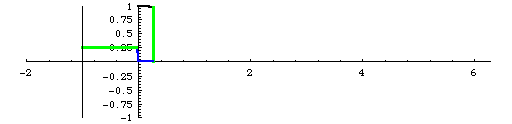# The Cosine CurveBlue Length = Blue Length; Green Length = Green Length

The x-coordinate of the point on the unit circle determined by a central angle (in standard position) of x radians is Cos(x). In a unit circle, the radian measure of an angle is the length of the arc subtended by that angle. To the left of the y-axis, you see a unit circle being swept out, with the radian measure of the angle (arc length) shown in blue, and the cosine of that angle (the x-coordinate) shown in green. To the right of the y-axis, you see the points of the graph of y = Cos(x) being graphed in black. The angle x is shown in blue and the value of Cos(x) is shown in green.

### Other Animated Sites

The Sine Curve
The Area of a Circle
The Area of a Circle II
The Pythagorean Theorem

WKU Mathematics Department .|. WKU Online

Last updated, Jan. 29, 2008
Tom.Richmond@WKU.edu